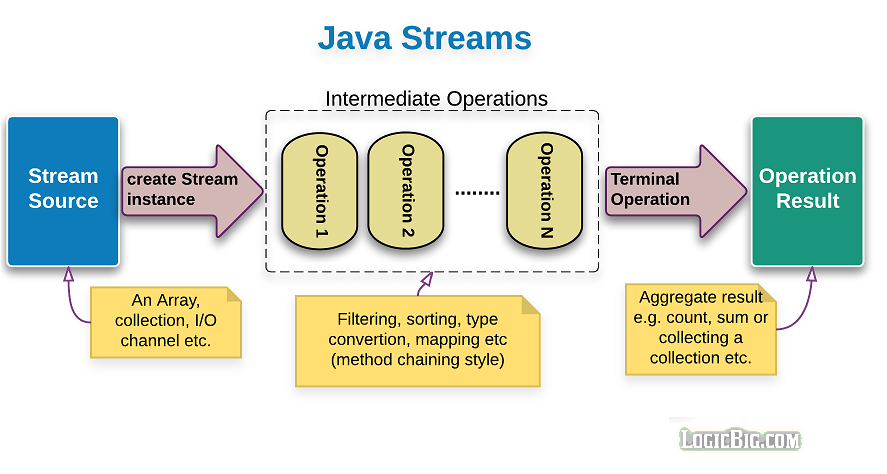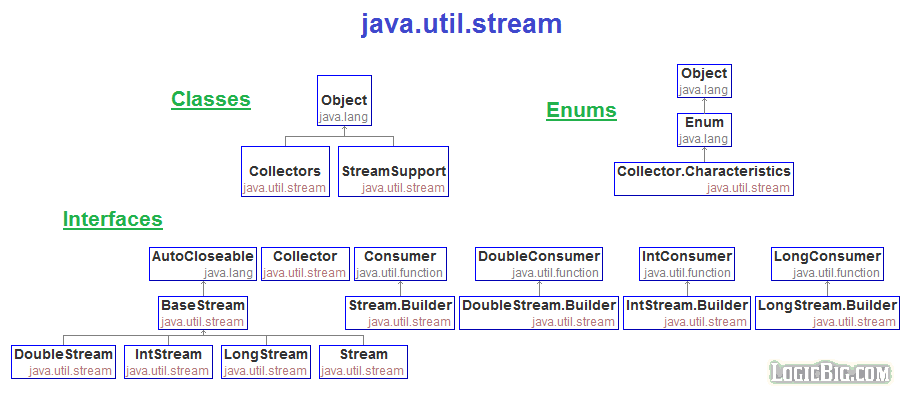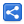# What are Java 8 streams?

[Last Updated: Feb 7, 2017]

Java stream API defines functional-style operations on discrete sequence of data elements. These data elements are usually provided by some standard data structures (ADT), for example the ones provided in java.util.collection. These data structures are typically called stream sources.

Java 8 enhanced the existing API like collections, arrays etc to add new methods to create stream object instances. This API itself provides some static method to generate finite/infinite stream of data elements.

Streams are functional, they operate on the provided source and produce results rather than modifying the source.

## Stream operations

A stream life cycle can be divided into three types of operation:

1. Obtaining the instance of Stream from a source. A source might be an array, a collection, a generator function, an I/O channel, etc
2. Zero or more intermediate operations which transform a stream into another stream, such as filtering, sorting, element transformation (mapping)
3. A terminal operation which produces a result, such as count, sum or a new collection.In this series of tutorials we will be exploring stream operations and the characteristics associated with them.

## What is Stream Pipeline?

A stream pipeline is nothing but combined intermediate and terminal operations

Many stream operations return a stream themselves. This allows operations to be chained to form a larger pipeline. This enables certain optimizations, such as laziness and short-circuiting, which we explore later.

According to the API docs:

Stream operations are divided into intermediate and terminal operations, and are combined to form stream pipelines. A stream pipeline consists of a source (such as a Collection, an array, a generator function, or an I/O channel); followed by zero or more intermediate operations such as Stream.filter or Stream.map; and a terminal operation such as Stream.forEach or Stream.reduce.

## java.util.stream API## Obtaining stream instances from the source

Java 8 has modified the existing collection and other data structures API to create/generate stream instances (please see the next section on stream instance). For example:

• Collection interface has added new default methods like `stream()` and `parallelStream()` which return a Stream instance.

```        List<String> list = Arrays.asList("1", "2", "3");
Stream<String> stream = list.stream();```

• New method which generate stream instances have been added in Arrays class.

```        String[] strs = {"1", "2", "3"};
Stream<String> stream = Arrays.stream(strs);```

## The stream interfaces

This API defines Stream interface for object elements. For primitives it defines IntStream, LongStream and DoubleStream interfaces. For example if we modify the above code to define array of int rather than array of String:

```        int[] ints = {1, 2, 3};
IntStream stream = Arrays.stream(ints);```

The extra primitive streams are provided for efficiency because wrapping primitives to Number objects and auto-boxing are relatively costly process.

The methods define by these interfaces can be divided into following two categories:

• Intermediate operations:
• These methods usually accept functional interfaces as parameters and always return a new stream.

Functional interface parameter!! that means, We can take full advantage of lambda expressions here.

In fact using lambda expressions which can participate an 'internal iteration', was the sole motivation for introducing this API.

#### What is internal iteration:

The internal iterator, is kind of iterator where the library API takes care of iteration rather than application code has to do iteration itself (external iterator).

• These methods store the provided functions rather than evaluating them at the same time, associate them with 'this' stream instance for later use (at the actual traversal step in the terminal method) and then return a new instance of the Stream

• Terminal operations: These methods produce some result e.g. `count()`, `max(..)`, `toArray(..)`, `collect(..)` .

## Imperative vs Declarative styles of coding

In imperative programming, we have to write code line by line to give instructions to the computer about what we want to do to achieve a result. For example iterating through a collection of integer using 'for loop' to calculate sum is an imperative style. We have to create local variables and maintain the loop ourselves rather than focusing on what result we want to achieve. It's like repeating the same logic regarding looping and calculating the sum every time.

In declarative programming, we just have to focus on what we want to achieve without repeating the low level logic (like looping) every time. Stream API achieve this by using internal iterator constructs along with lambda expressions. They not only take care of iteration but also provide intermediate and terminal operations to customize the outcome, and additionally abstract away, the well-tested algorithms and parallelism, hence giving the best performance.

## Examples

In following examples we will compare the old imperative style with new declarative style.

Old Imperative Style New Declarative Style
Internal iteration, using new default method `Iterable#forEach(Consumer<? super T> action)`
```List<String> list =
Arrays.asList("Apple", "Orange", "Banana");
for (String s : list) {
System.out.println(s);
}```
```List<String> list =
Arrays.asList("Apple", "Orange", "Banana");
//using lambda expression
list.forEach(s -> System.out.println(s));
//or using method reference on System.out instance
list.forEach(System.out::println);```
Counting even numbers in a list, using `Collection#stream()` and `java.util.stream.Stream`
```List<Integer> list =
Arrays.asList(3, 2, 12, 5, 6, 11, 13);
int count = 0;
for (Integer i : list) {
if (i % 2 == 0) {
count++;
}
}
System.out.println(count);```
```List<Integer> list =
Arrays.asList(3, 2, 12, 5, 6, 11, 13);
long count = list.stream()
.filter(i -> i % 2 == 0)
.count();
System.out.println(count);```
Retrieving even number list
```List<Integer> list =
Arrays.asList(3, 2, 12, 5, 6, 11, 13);
List<Integer> evenList = new ArrayList<>();
for (Integer i : list) {
if (i % 2 == 0) {
}
}
System.out.println(evenList);```
```List<Integer> list =
Arrays.asList(3, 2, 12, 5, 6, 11, 13);
List<Integer> evenList =
list.stream()
.filter(i -> i % 2 == 0)
.collect(Collectors.toList());
System.out.println(evenList);```
##### Or if we are only interested in printing:
```List<Integer> list =
Arrays.asList(3, 2, 12, 5, 6, 11, 13);
list.stream().filter(i -> i % 2 == 0)
.forEach(System.out::println);```
Finding sum of all even numbers
```List<Integer> list =
Arrays.asList(3, 2, 12, 5, 6, 11, 13);
int sum = 0;
for (Integer i : list) {
if (i % 2 == 0) {
sum += i;
}
}
System.out.println(sum);```
```List<Integer> list =
Arrays.asList(3, 2, 12, 5, 6, 11, 13);
int sum = list.stream()
.filter(i -> i % 2 == 0)
.mapToInt(Integer::intValue)
.sum();
System.out.println(sum);```
##### Alternatively
```List<Integer> list =
Arrays.asList(3, 2, 12, 5, 6, 11, 13);
int sum = list.stream()
.filter(i -> i % 2 == 0)
.reduce(0, (i, c) -> i + c);
System.out.println(sum);```
Finding whether all integers are less than 10 in the list
```List<Integer> list =
Arrays.asList(3, 2, 12, 5, 6, 11, 13);
boolean b = true;
for (Integer i : list) {
if (i >= 10) {
b = false;
break;
}
}
System.out.println(b);```
```List<Integer> list =
Arrays.asList(3, 2, 12, 5, 6, 11, 13);
boolean b = list.stream()
.allMatch(i -> i < 10);
System.out.println(b);```

Also look at `Stream#anyMatch(...)` method

Finding all sub-directory names in a directory. Using new static methods, `Arrays#stream(T[] array)`
```List<String> allDirNames = new ArrayList<>();
for (File file : new File("d:\\").listFiles()) {
if(file.isDirectory()){
}
}
System.out.println(allDirNames);```
```List<String> allDirNames =
Arrays.stream(new File("d:\\")
.listFiles())
.filter(File::isDirectory)
.map(File::getName)
.collect(Collectors.toList());
System.out.println(allDirNames);```

 Share